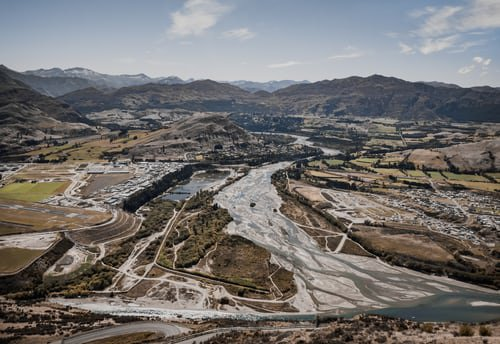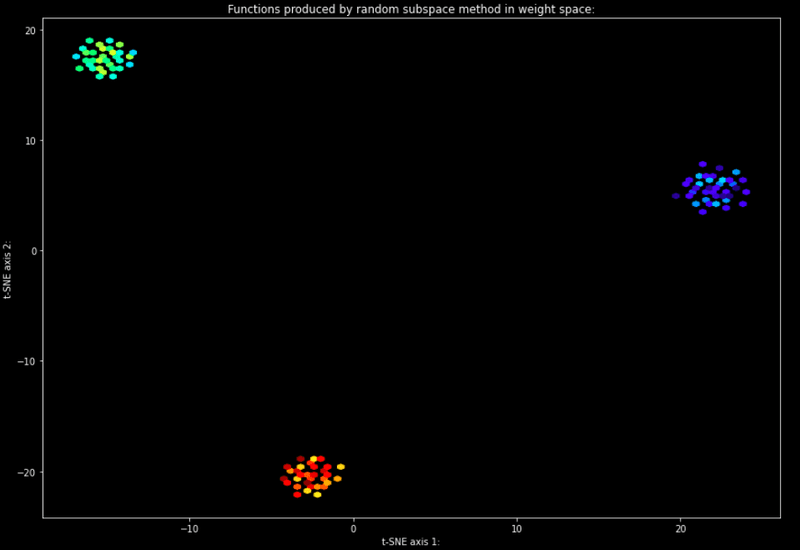Machine Learning

#### Why Deep Ensembles Are So Effective: A Loss Landscape Perspective

It is well known that forming an ensemble from deep neural networks is a reliable approach to improving generalisation performance. In this article, we will cover and reproduce a set of experiments in an attempt to gain a level of intuition around why this phenomenon occurs.A landscape of local minima (valleys) and local maxima (peaks)

It is well known that forming an ensemble from deep neural networks is a reliable approach to improving generalization performance. However, these results have been shown to be empirically true. As a result, there is no universally accepted explanation for the efficacy of this technique.

In this article, we will cover and reproduce a set of experiments defined in the research paper, Deep Ensembles: A Loss Landscape Perspective, that provides an explanation as to why deep ensembles are so effective.

### Experimental Setup

The MNIST dataset was used in the following experiments. Each of the models used to fit the data was a simple multi-layer perceptron.

In order to conduct the following experiments, three models were trained and then compared.

If you would like to reproduce the results yourself, I have made the source code for the experiments available here.

## Deep Ensembles vs. Subspace Sampling Methods

Before we proceed to the experiments, it is important that the difference between deep ensembles and subspace sampling techniques is well understood.

A deep ensemble is an ensemble of neural networks. Each of these neural networks is independent of the other neural networks in the ensemble. That is, the parameter vector for each model is attained independently from the parameter vector of any other model in the ensemble.

In contrast, subspace sampling techniques derive the parameter vector for each model in the ensemble from one original parameter vector. In order to create an ensemble using a subspace sampling technique, a single model is trained and then used to create the other models for the ensemble.

## Visualizing Function Space Similarity

In order to gain some intuition as to why deep ensembles outperform subspace sampling techniques, we will compare the models produced by each of the techniques.

We will begin by comparing how a single model evolves throughout the training process independently.

Following this, we will compare the trajectories through function space followed by each model.

### The similarity of Functions Within a Single Randomly Initialised Trajectory

We will compare the similarity between successive checkpoints along a single trajectory.

In order to compute the similarity between two models, we will compute the cosine similarity between the parameter vectors of the models.

The results of this comparison for the three models are as follows:

As expected at the beginning of training, the parameter vectors between successive epochs differ greatly. After a few epochs of training, the models begin to converge.

The models were trained using the Adam optimizer and a fixed learning rate. As a result of this, the models were not able to fully converge to the local optima but oscillate around it. This can be seen in the later epochs of training as represented by the repeated pattern show in the plot.

### Prediction Agreement of Functions Within a Single Randomly Initialised Trajectory

We will compare the proportion of the samples that are predicted to have the same label between two successive checkpoints in training.

It can be seen that at the beginning of the training process, the predictions produced by each model vary drastically between successive epochs. However, in the later epochs, the models begin to converge and produce very similar predictions between successive epochs.

Once again, the oscillation of the models around the local minima is represented by the repeated pattern shown in the later epochs of training.

### Trajectories of Models Through Weight Space

We will now attempt to visualize how each of the models moves through weight space during training. This will be done by projecting the parameter vector of each model at each epoch of training into two dimensions and then plotting these results.

Firstly, t-SNE will be used to project the parameter vectors into two dimensions. The results are as follows:

The colors represent the epoch of training: blue represents the start of training and red represents the end of training.

Secondly, PCA will be used to project the parameter vectors into two dimensions. The results are as follows:

### Comparing the Similarity of Functions Across Randomly Initialised Trajectories

Having seen how each of the models evolved throughout the training process individually, we can now compare the models to one another.

We will begin by comparing the trajectories followed by each of the models through weight space during the training process.

The t-SNE projection results are as follows:

The PCA projection results are as follows:

A key observation to be made here is the distance between each of the models in weight space. This is believed to be a key contributing factor to the increased performance provided by deep ensembles when compared to subspace sampling techniques. This will be explained in more detail later.

## Comparison of Deep Ensembles and Subspace Sampling Techniques in Function Space

Now that we have seen that each of the models in a deep ensemble differs greatly, we will analyze the similarity between each of the models in an ensemble produced by a subspace sampling technique.

The random subspace sampling technique will be analyzed here. However, other methods have been shown to behave similarly in the original paper.

To analyze the similarity between each of the models in the ensemble, we will once again make use of dimensionality reduction to plot the parameter vectors associated with each model in the ensemble.

In the following plot, the final parameter vector with each of the trained models was used with the random subspace sampling technique to produce an ensemble. Each of the models in a given ensemble have been given the same color. t-SNE was used to project the parameter vectors into two dimensions.Ensembles produced by random subspace sampling

It can be seen that the subspace sampling technique produces a set of similar models. This is in contrast to the set of highly diverse models in a deep ensemble.

This is believed to be the reason for the superior performance of deep ensembles in comparison to subspace sampling techniques.

In deep ensembles, the trajectories of randomly initialized neural networks explore different modes in function space, whereas subspace sampling techniques tend to focus on a single mode.

## Conclusion

If you have not already, I highly recommend that you read the original research paper. The paper not only contains numerous experiments and results that were not included here but also analyses much larger networks that are closer to what would be seen in practice.

##### Enjoyed this read?

Stay up to date with the latest AI news, strategies, and insights sent straight to your inbox!

Thank you! Your submission has been received!
Oops! Something went wrong while submitting the form.